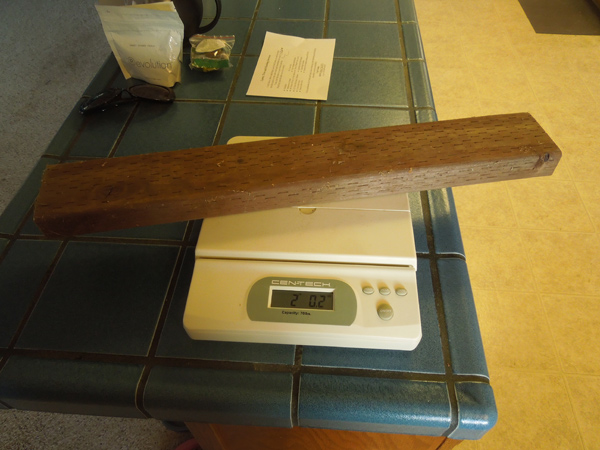# Weighing a two by four on the scale

Forget estimates. This is the real weight of a two by four.

How Much Does a two by four Weigh?# two by four: 2 lbs., 0 oz.

## Description

• A two foot section of pressure-treated 2x4.
• this two-foot section weighed almost exactly two pounds, figure one pound per foot.

## Weight

• Imperial: 2 lbs., 0 oz.
• Metric: 0.91 kg. ( 910 grams )

Back to the Complete List of Weight Tests >

Weight of these items was actually tested. Weight will vary, but these are the actual measurements.